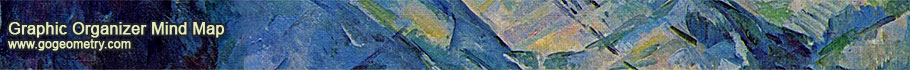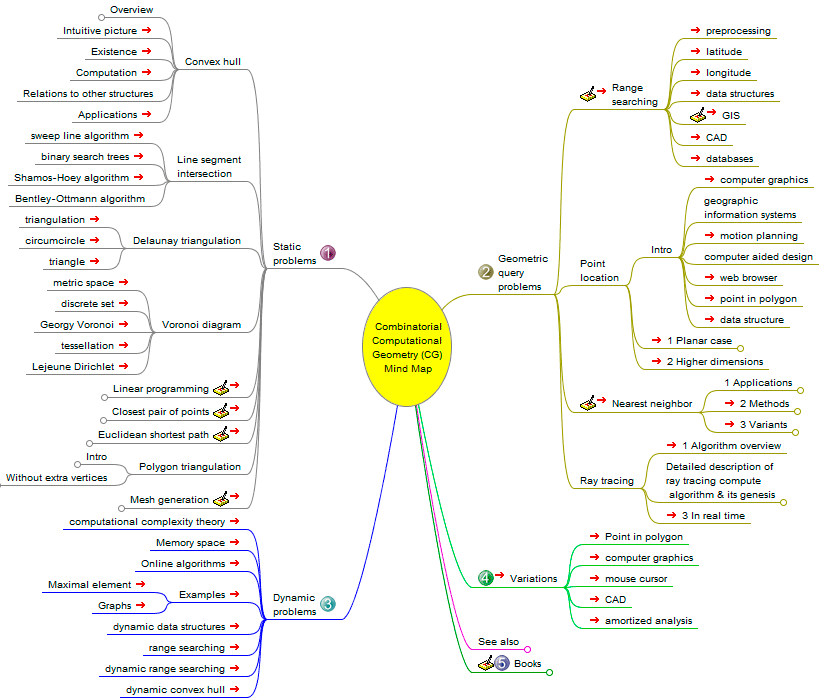# Combinatorial Computational Geometry, Mind Map and NewsCombinatorial Computational Geometry
The primary goal of research in combinatorial computational geometry is to develop efficient algorithms and data structures for solving problems stated in terms of basic geometrical objects: points, line segments, polygons, polyhedra, etc.

The following general problem classes may be distinguished:

• Static problems

• Geometric query problems

• Dynamic problems

• Variations

Computational Geometry
Computational geometry is a branch of computer science devoted to the study of algorithms which can be stated in terms of geometry. Some purely geometrical problems arise out of the study of computational geometric algorithms, and such problems are also considered to be part of computational geometry.

The main impetus for the development of computational geometry as a discipline was progress in computer graphics and computer-aided design and manufacturing (CAD/CAM), but many problems in computational geometry are classical in nature, and may come from mathematical visualization.

The main branches of computational geometry are: (1) Combinatorial computational geometry, also called algorithmic geometry and (2) Numerical computational geometry, also called machine geometry, computer-aided geometric design (CAGD), or geometric modeling. Source: Wikipedia, Computational geometry.

Graphic organizers
Graphic organizers are visual representations of knowledge, concepts or ideas.Home | Search | Mind Maps | Software | Computational Geometry | Post a comment | Email